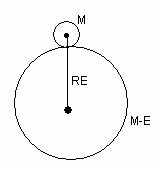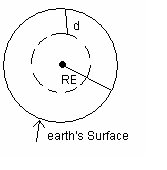# Acceleration due to gravity

## Acceleration due to gravity of earth

• Earth attracts every object lying an its surface towards its centre with a force known as gravitational towards its centre with a force known as gravitational pull or gravity.
• Whenever force acts on any body it produces acceleration and in case of gravitation this acceleration produced under effect of gravity is known as accelesation due to gravity (g)
• Value of accelesation due to gravity is independent of mass of the body and its value near surface of earth is 9.8 ms-2
• Expression for acceleration due to gravity
Consider mass of earth to be as ME and its redius be RE Suppose a body of mass M (much smaller then that fo earth) is kept at the earth surface. Force eseerted by earth on the body of mass m is
$F=\frac{GMM_{E}}{R_{E}^{2}}$
The force for the body due to earth produces acceleration due to gravity
(g) in the motion of the body. From Newton's Second law of motion
$f=mg$
from above equations
$g=\frac{GM_{E}}{R_{E}^{2}}$
which is acceleration due to gravity at earth's surface.

## Acceleration due to gravity below and above the earth surface

(i)Above earth's surface• An object of mass m is placed at hight h above the earth's active in this object is
$F=\frac {-GMM_{E}}{(R_{E}+h)^{2}}$
From this it can be concluded that value of g decreases as distance above surface of earth increases now,
$g=\frac{GM_{E}}{R_{E}(1+\frac{h}{R_{E}})^{2}}$
$g=\frac{g_{0}}{(1+\frac{h}{R_{E}})^{2}}$
Where $g_{0}=\frac{GM_{E}}{R_{E}^{2}}$ value of acceleration due to gravity at earth surface
• for h<<R
$g=g_0(1+ \frac {h}{R_E})^{-2}$
Expanding by Binomial theriom
$g=g_0(1- \frac {2h}{R_E})$
• Above equation tells us that for small hight h above surface of earth. value of g decreases by factor $(1-\frac {2h}{R_E})$

(ii)Below the earth's surface• If one goes inside the earth surface the value of g again decreases
• $\rho$ = density of material of earth them
$m=\frac {4}{3}(R_E)^3 \rho$
From this acceleration due to gravity at earth's Surface is
$g_{0}=\frac{G(4/3)R_{E}^{3} \rho}{R_{E}^{2}}$
$g_0=\frac {4}{3}G R_E \rho$ ---(A)
• Let g acceleration due to gravity at depth D below earth's surface
Body at depth d will experience force only due to portion of reduce (RE-d) of earth's
outer spherical shell of thickness d will not exeperience any force
M is mass of the portion of earth with radius (RE-d) then
$g=\frac{GM}{r^{2}}$
$M=\frac {4}{3}(R_E-d)^3 \rho$
$g=\frac{G(4/3)(R_{E}-d)^{3}P}{(R_{E}-d)^{2}}$
$g= \frac {4}{3}G (R_E -d) \rho$ ---(B)
Dividing epn (B) by (A)
$\frac {g}{g_0}= (1- \frac {d}{R_E})$
or $g=g_0 (1- \frac {d}{R_E})$
• from above equation is clear that acceeleration due to gravity also decreases with depth.